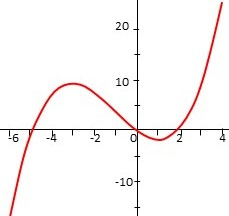# Consider the graph of y= f '(x)| shown below. Give the largest value of x| where the graph of...

## Question:

Consider the graph of y= f '(x)| shown below. Give the largest value of x| where the graph of f(x)| has a point of inflection.## Inflection Points:

The inflection points of a function are closely linked to the behavior of the first derivative:

at the local ends of the first derivative of the function, the inflection points of the function are reached.

## Answer and Explanation:

The largest value of the independent where the graph of the function has a point of inflection is 1, at this point the derivative of the function has a local extreme.

#### Learn more about this topic:Understanding Concavity and Inflection Points with Differentiation

from Math 104: Calculus

Chapter 10 / Lesson 6
14K